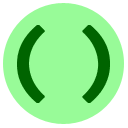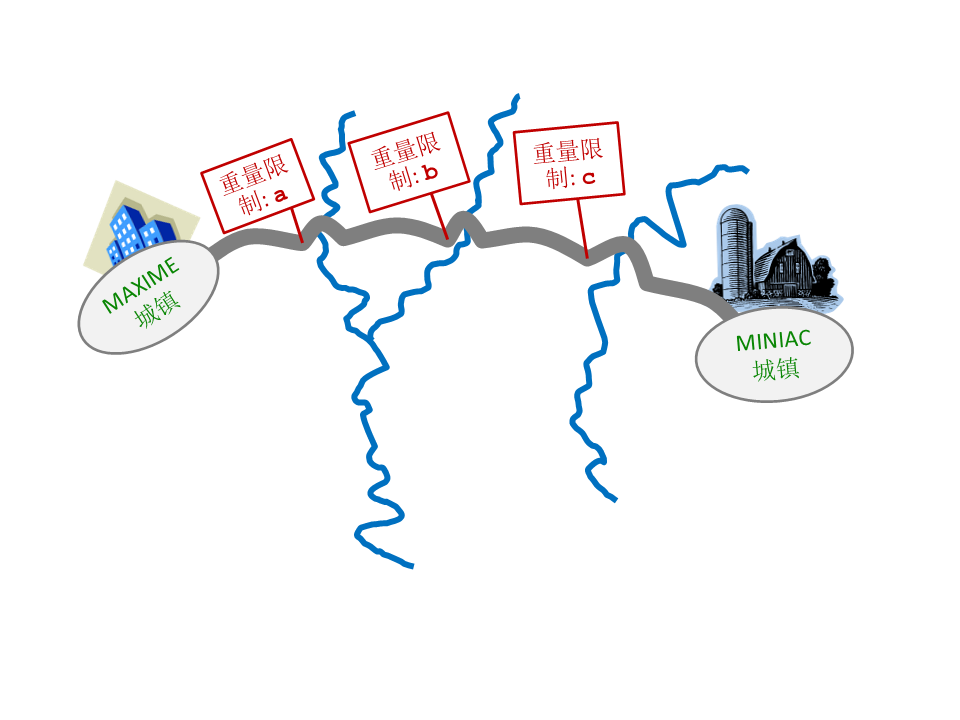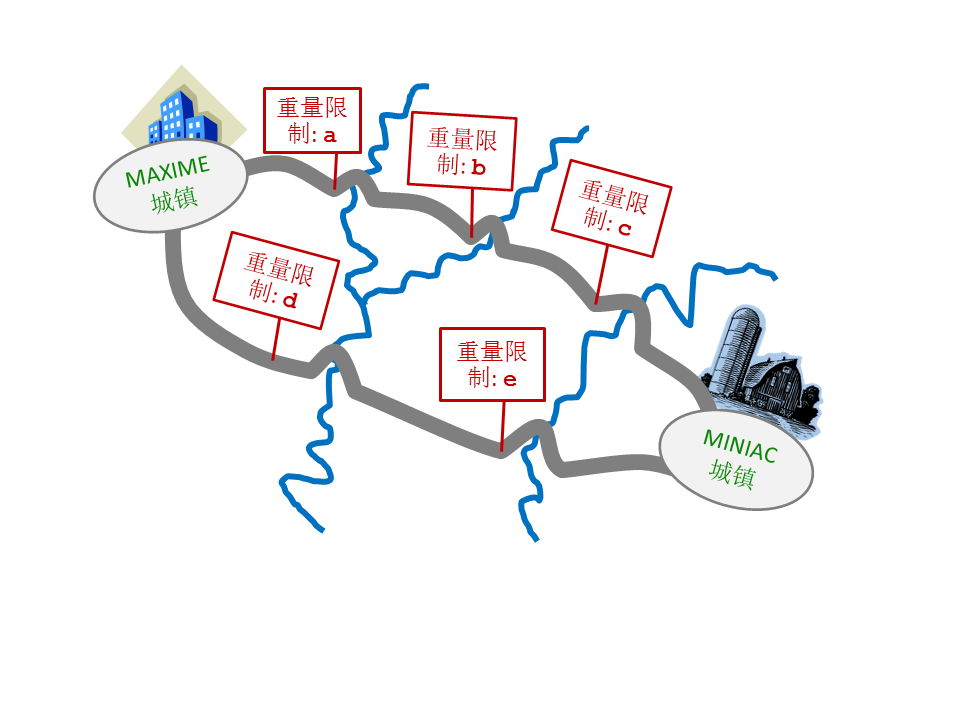# 2: 函数上面示例程序中多余的空格对输出并没有任何影响。多余的空格在其他大多数情况下也都是无意义的。不过，要小心每一行开始时的额外空格，他们叫做缩进,并且有一个特殊的意义。当你不正确的使用时，缩进可能会造成错误（点击链接来看一个示例）。你将会在几节课后学到怎样正确的使用缩进。<pre>x = 13
y = 7
a = max(x+y, x*2)
b = min(x, y)
print(a,b)</pre><pre>x = min(max(13, 7), 9)
print(x)</pre>

## 常见错误

Python说这里有一个语法错误，也就是说它无法理解你想干什么：

``` Traceback (most recent call last):
In line 2 of the code you submitted:
bigger = max(3, 4)
^
语法错误：无效的语法```

## 练习## 练习：代码排序• print(max(x, y, z))
• print(min(x, y, z))
• print(x+y+z-min(x, y, z)-max(x, y, z))

• 你可以直接进入第三课，或者
• 你可以在课程2X中做一些关于函数的额外练习。